# RD Sharma Solutions For Class 7 Maths Exercise 20.2 Chapter 20 Mensuration - I (Perimeter and area of rectilinear figures)

The PDF of RD Sharma Solutions for Class 7 Maths Chapter 20 Mensuration – I (Perimeter and Area of rectilinear figures) Exercise 20.2 are provided here. Students who are preparing for their exams and who wish to present their answers without any mistakes can refer to RD Sharma Solutions for Class 7. On regular practice, students can speed up the method of solving problems by using short-cut tips to secure high marks in their examination. Students can download the pdf from the links provided below. This exercise deals with the area between two rectangles.

## Download the PDF of RD Sharma Solutions For Class 7 Chapter 20 – Mensuration – I (Perimeter and Area of rectilinear figures) Exercise 20.2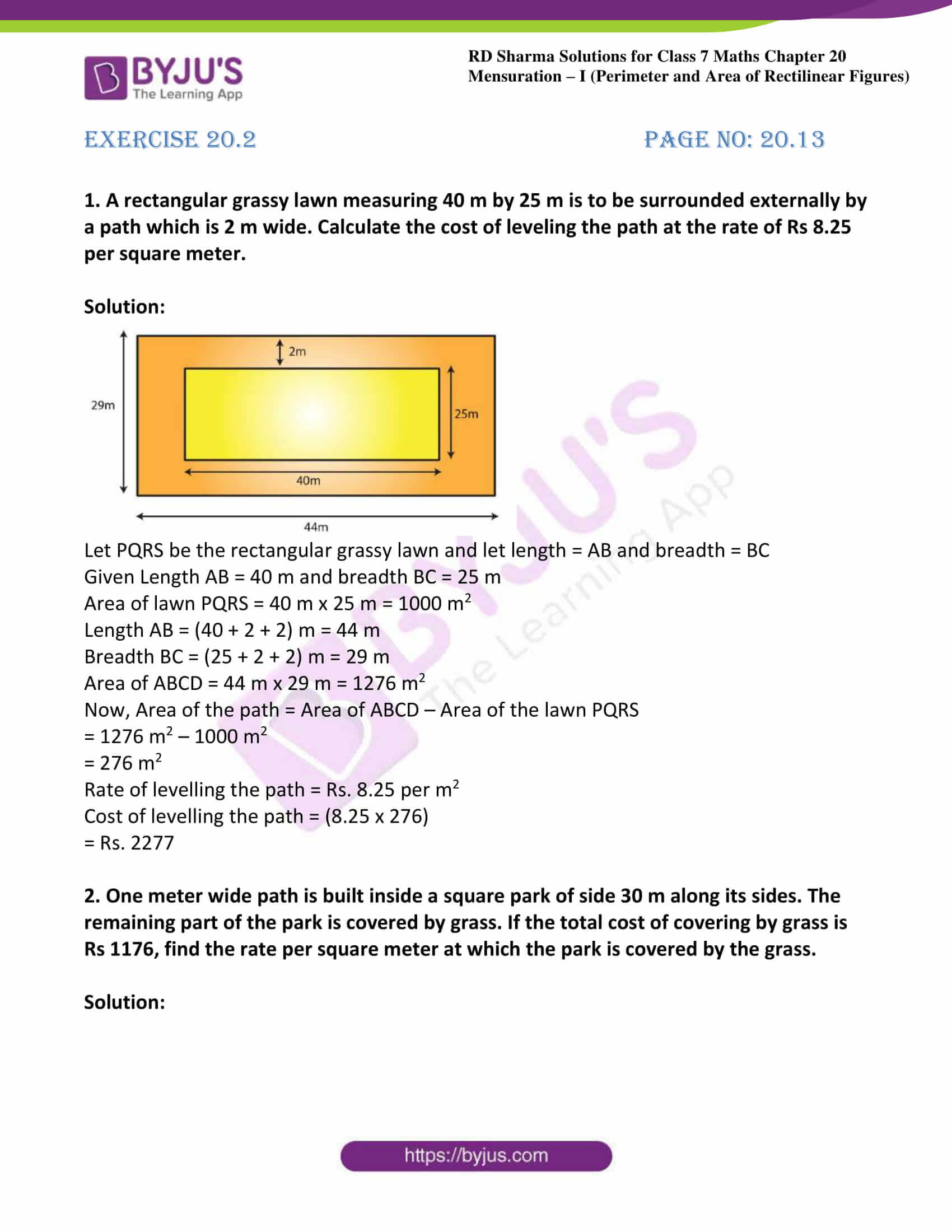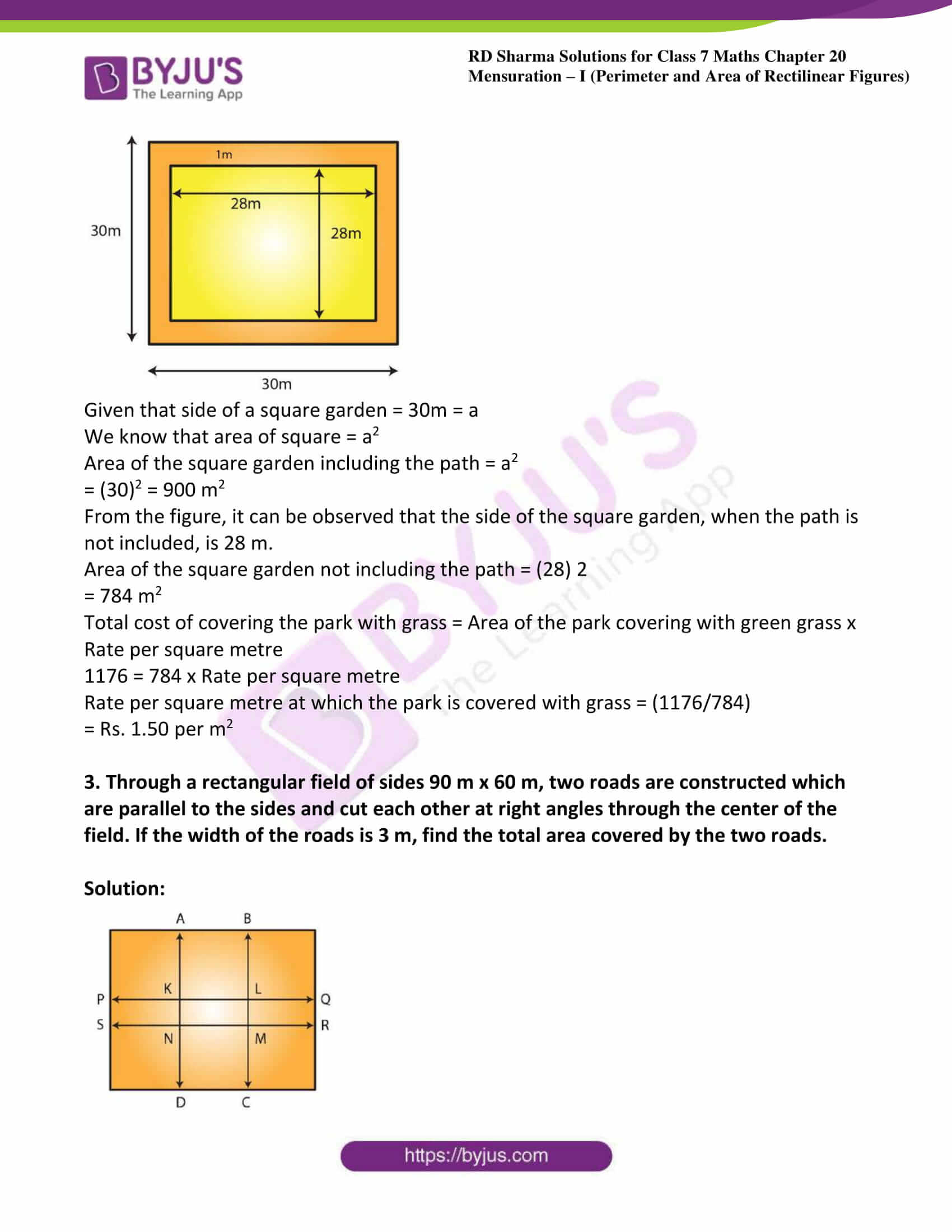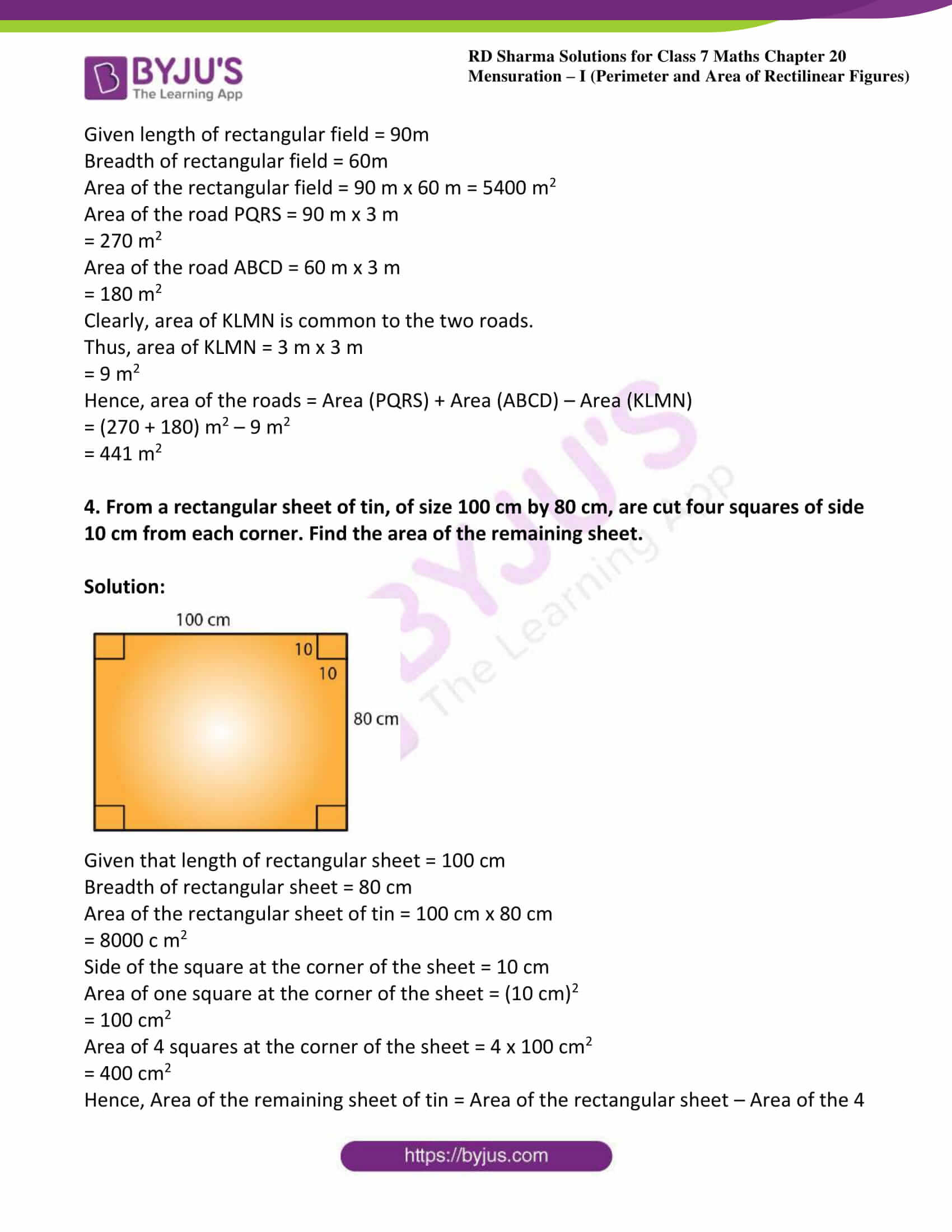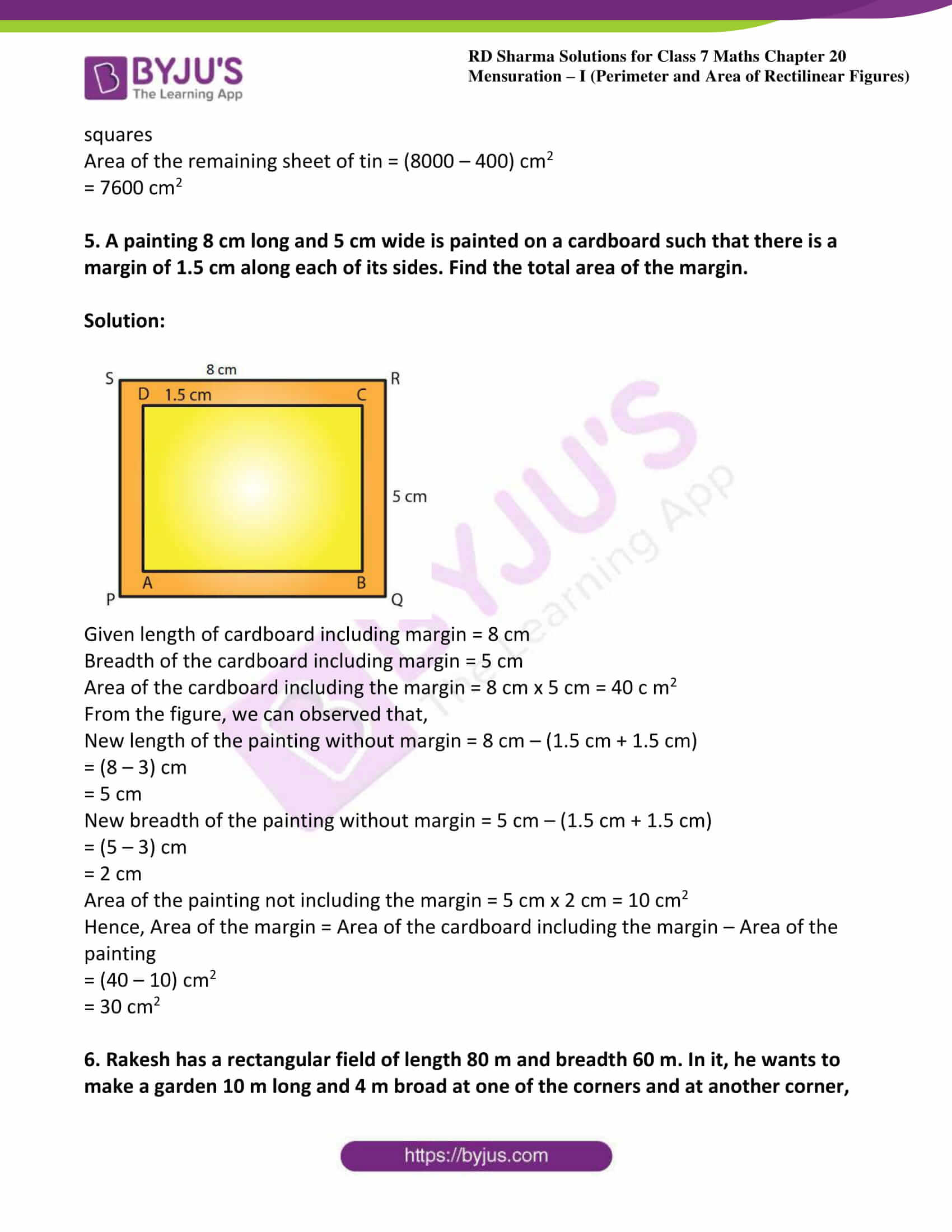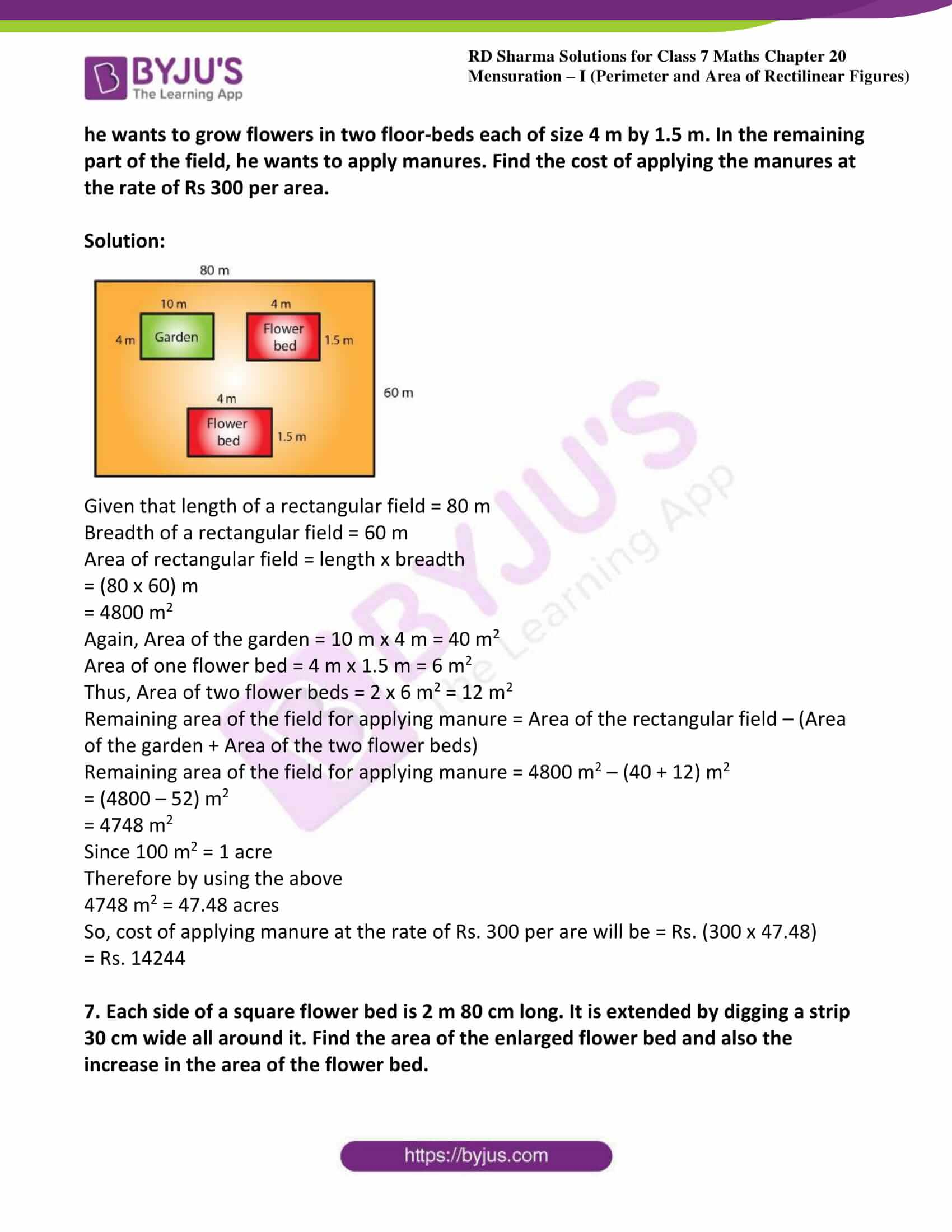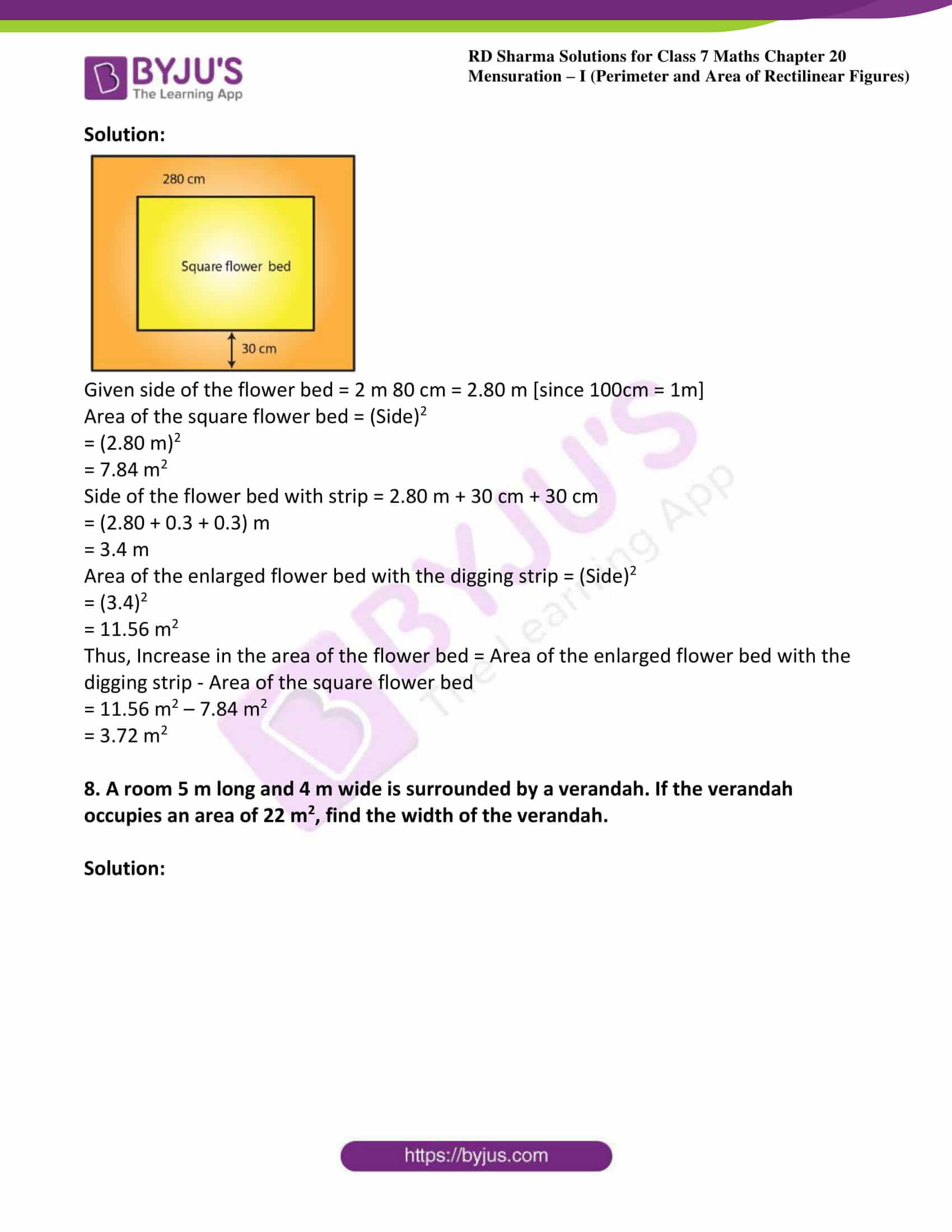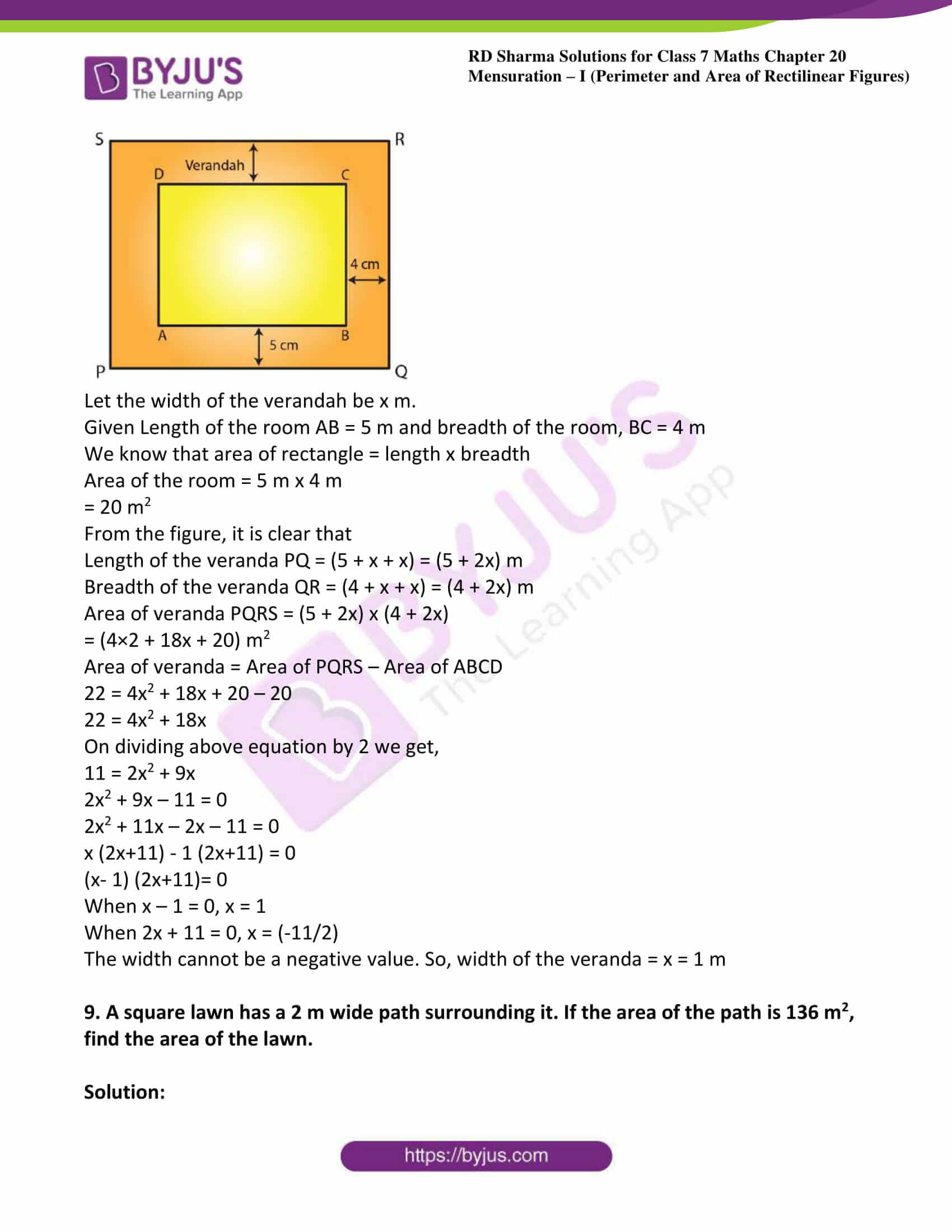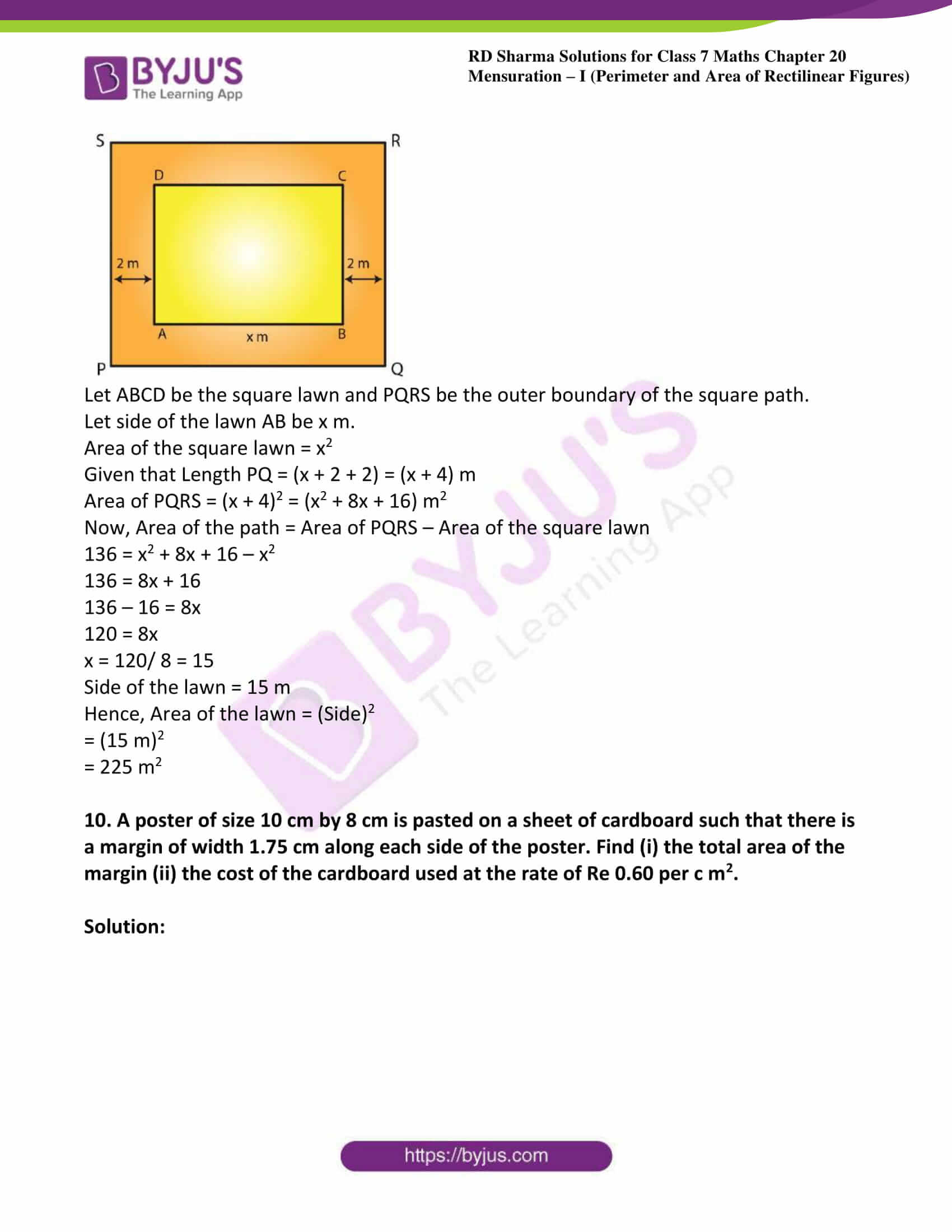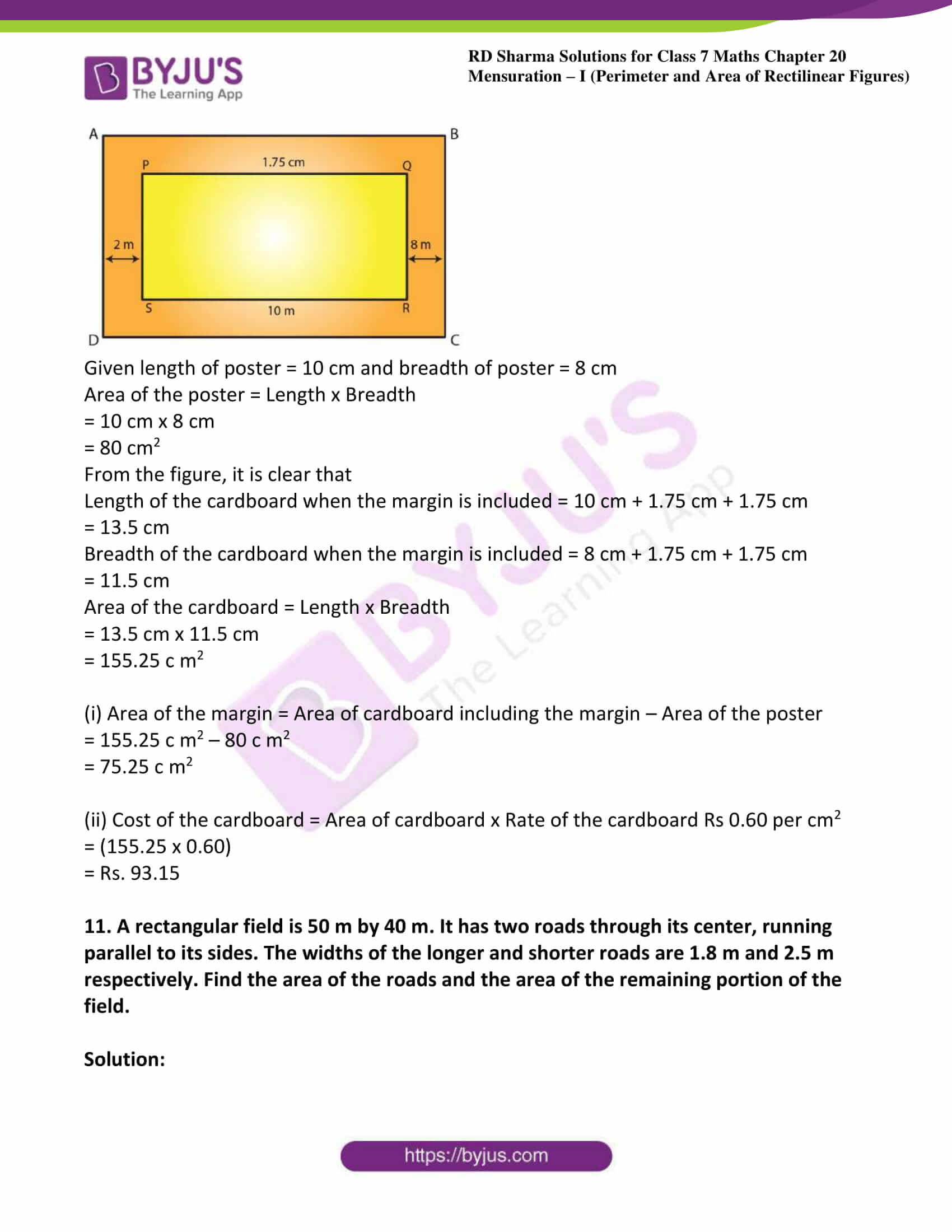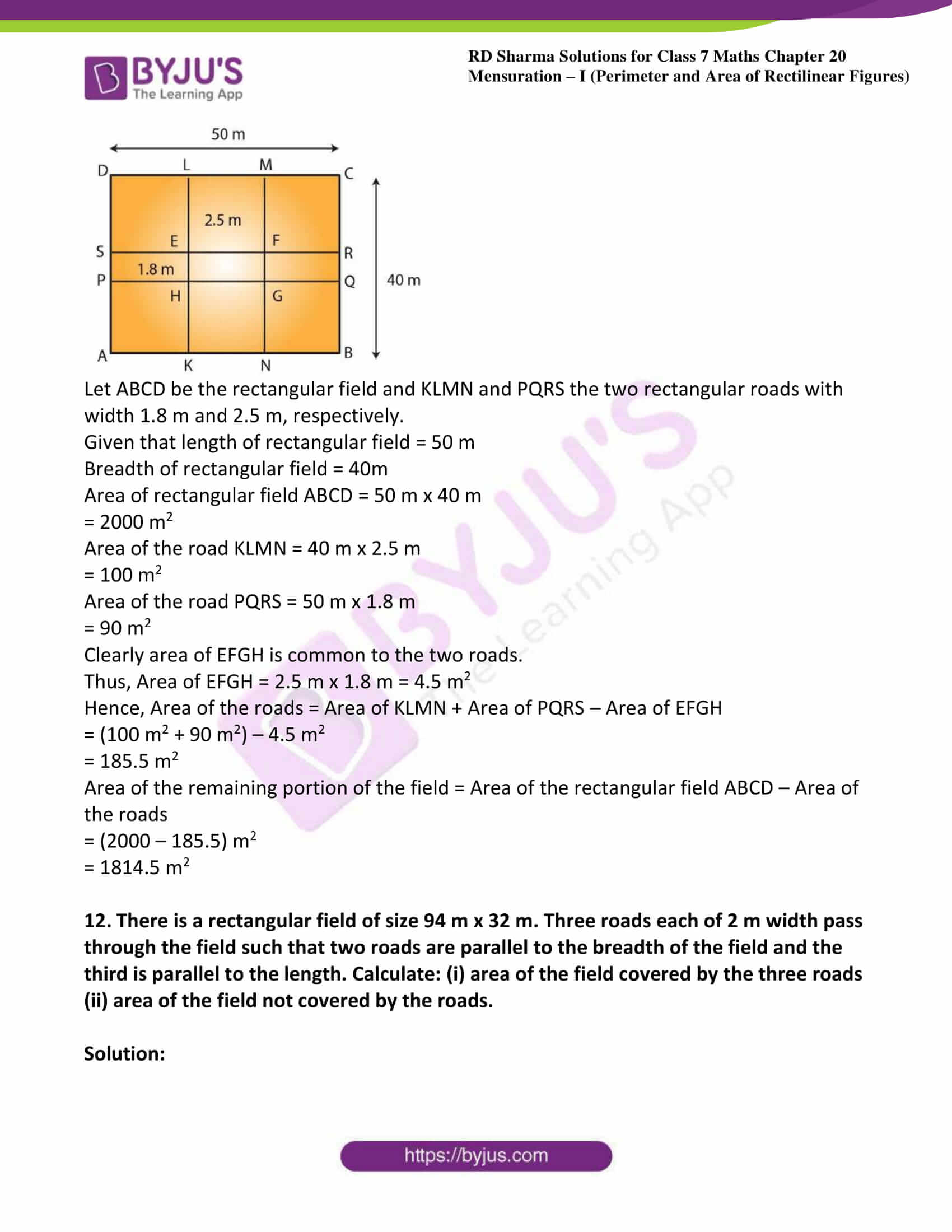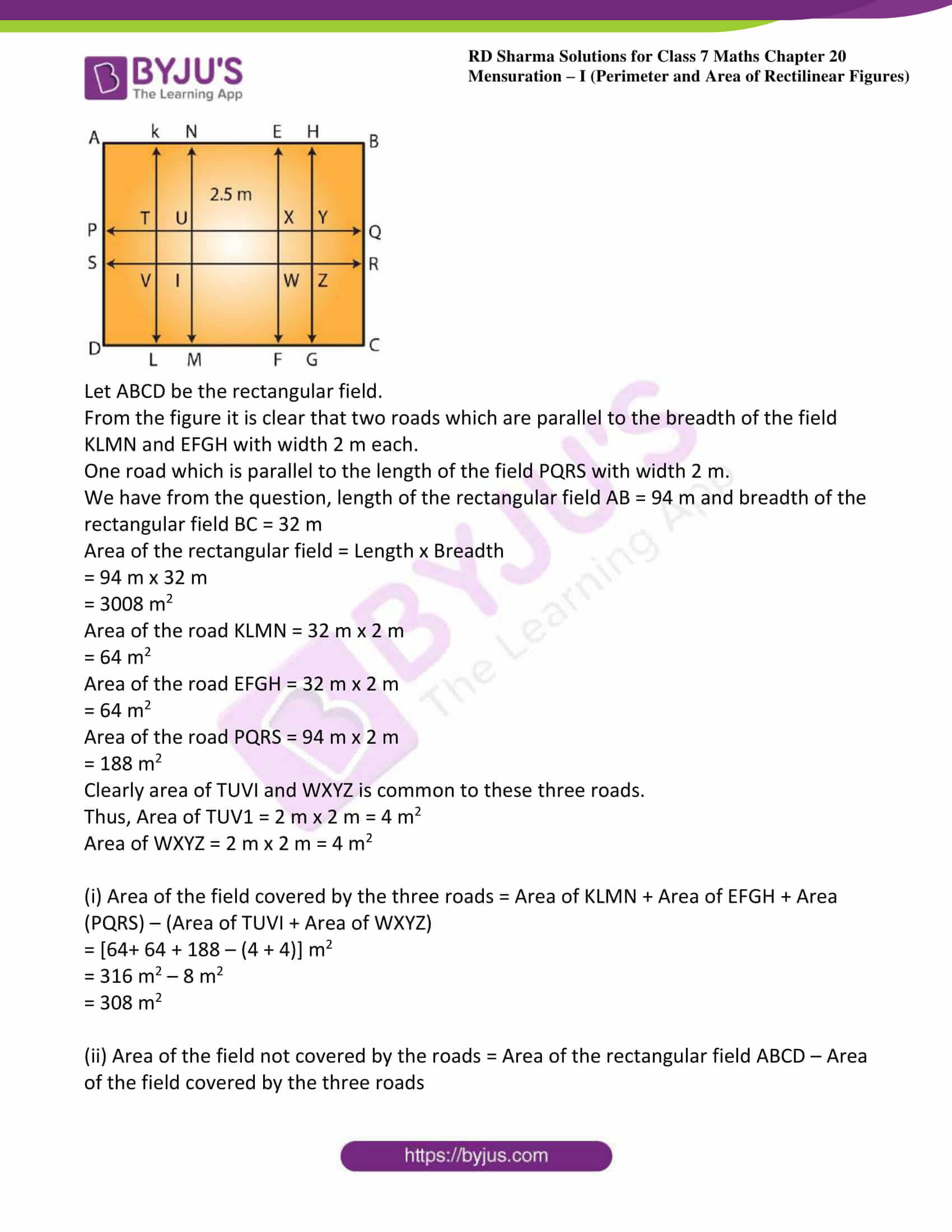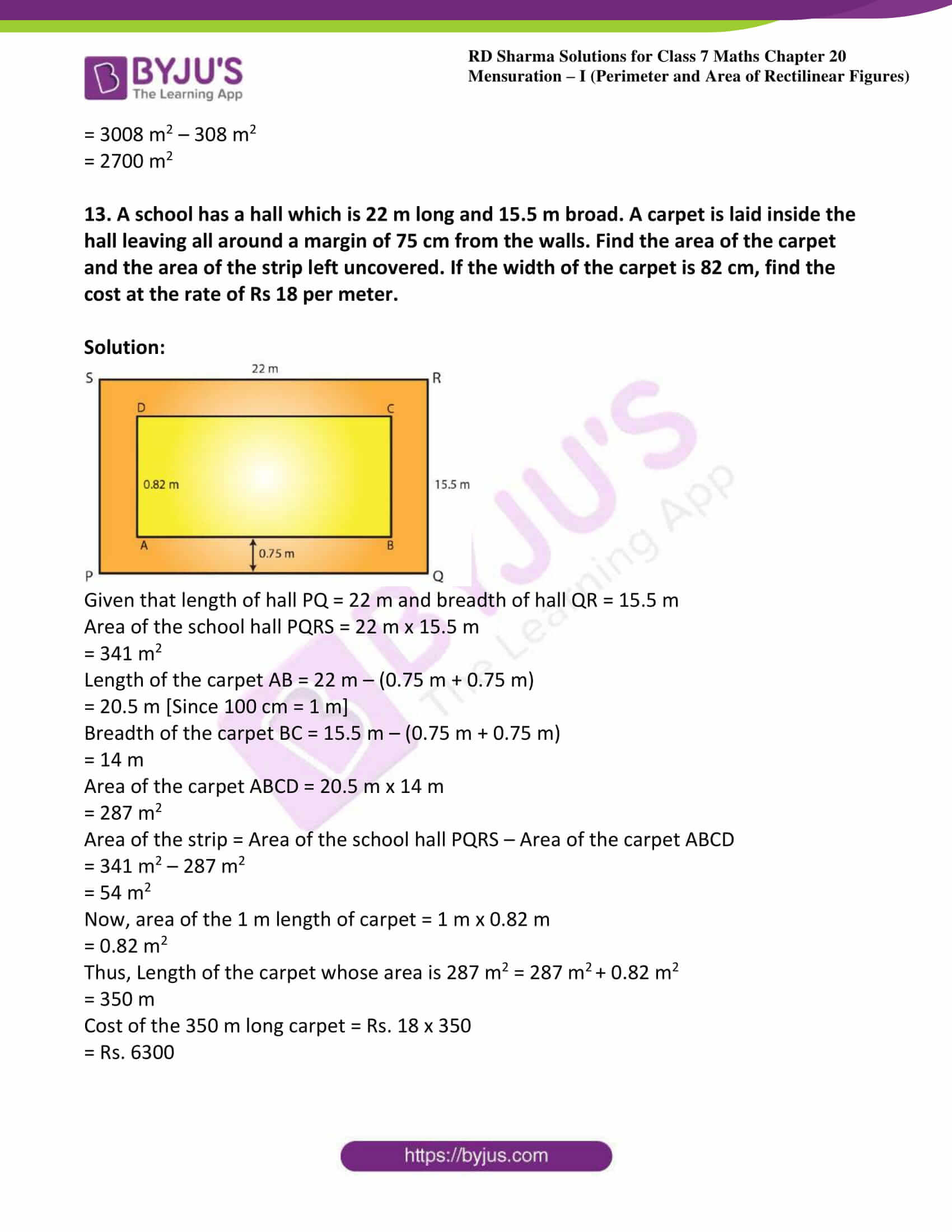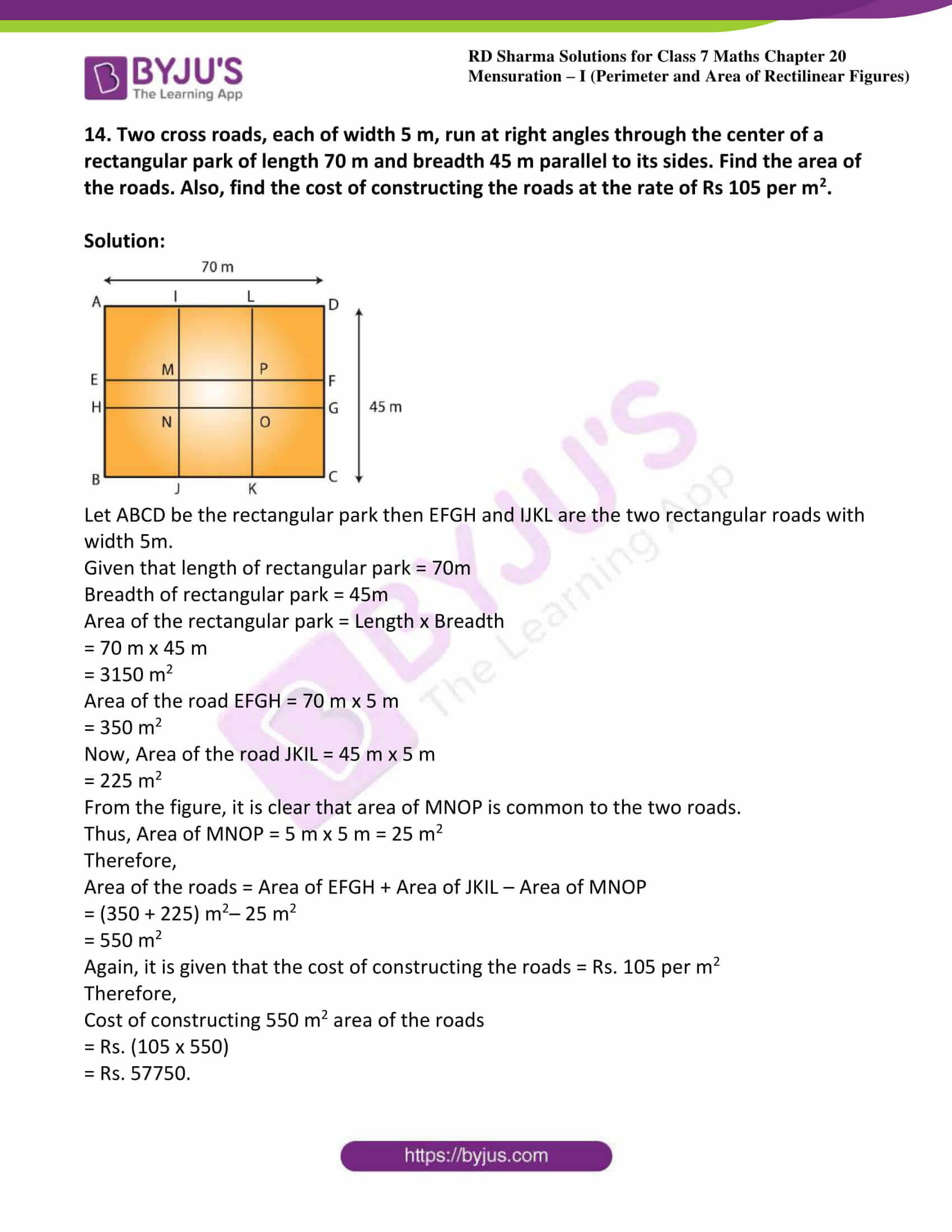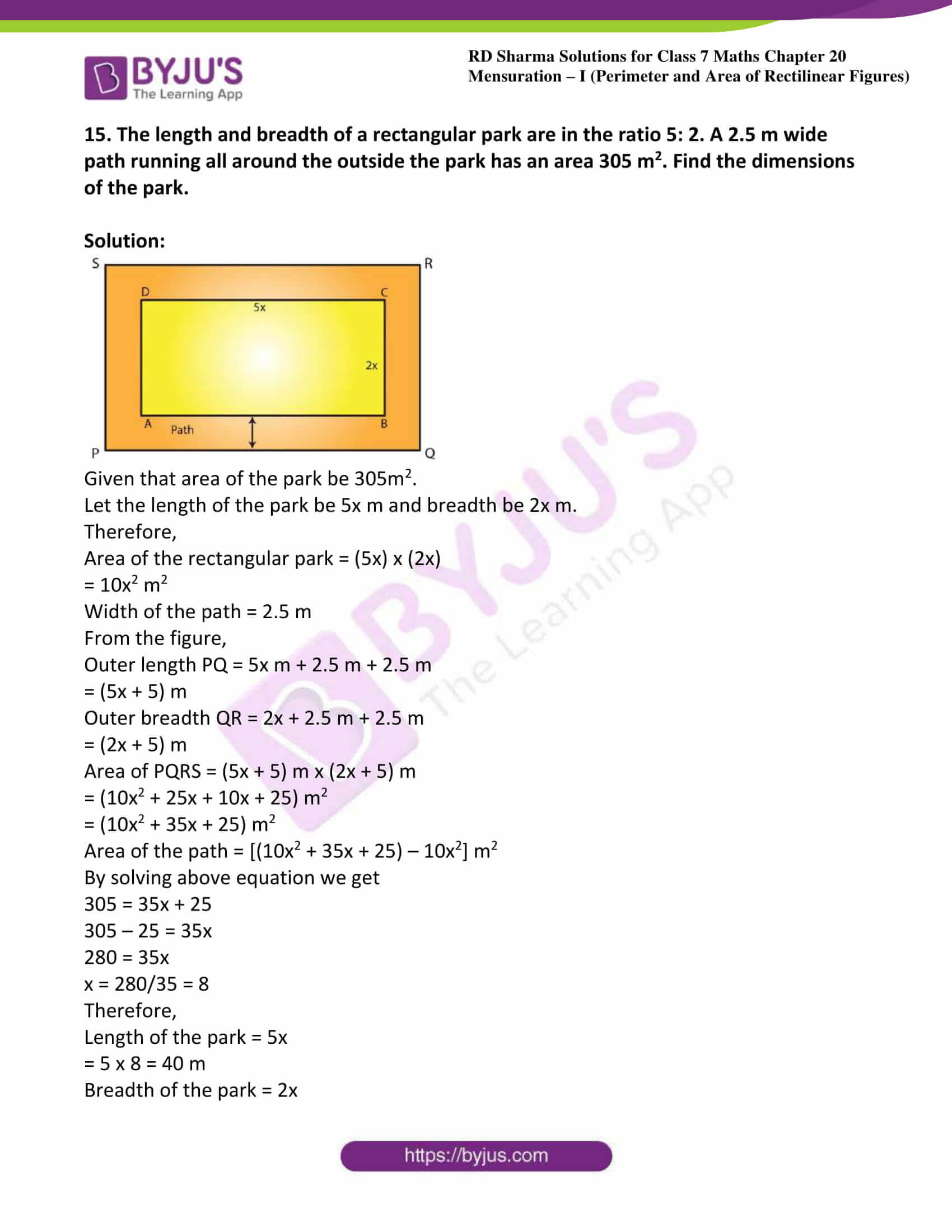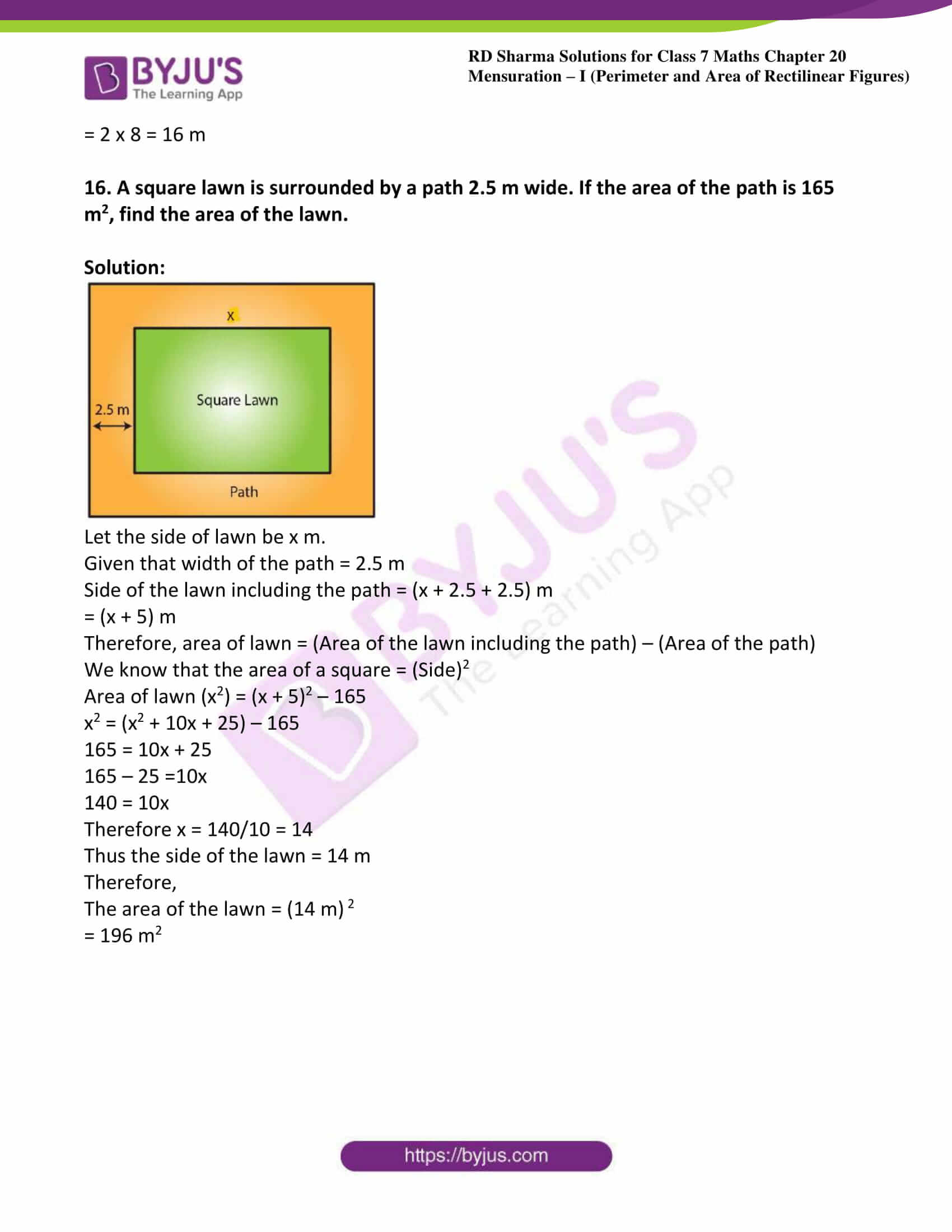### Access answers to Maths RD Sharma Solutions For Class 7 Chapter 20 – Mensuration – I (Perimeter and Area of rectilinear figures) Exercise 20.2

1. A rectangular grassy lawn measuring 40 m by 25 m is to be surrounded externally by a path which is 2 m wide. Calculate the cost of leveling the path at the rate of Rs 8.25 per square meter.

Solution: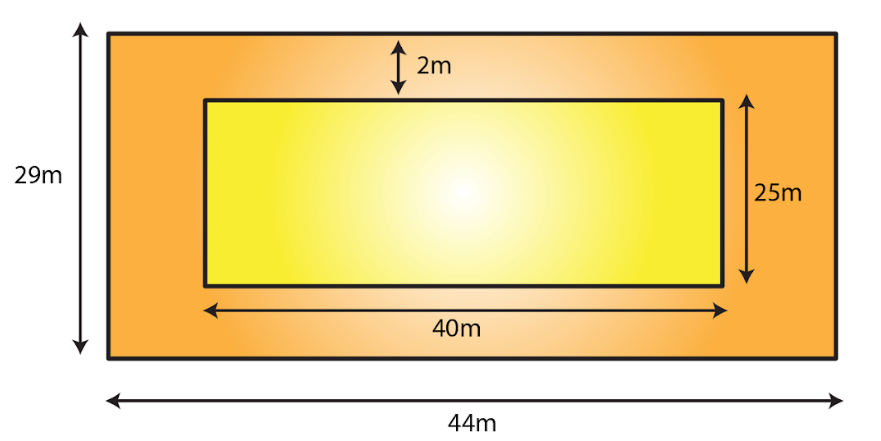Let PQRS be the rectangular grassy lawn and let length = AB and breadth = BC

Given Length AB = 40 m and breadth BC = 25 m

Area of lawn PQRS = 40 m x 25 m = 1000 m2

Length AB = (40 + 2 + 2) m = 44 m

Breadth BC = (25 + 2 + 2) m = 29 m

Area of ABCD = 44 m x 29 m = 1276 m2

Now, Area of the path = Area of ABCD – Area of the lawn PQRS

= 1276 m2 – 1000 m2

= 276 m2

Rate of levelling the path = Rs. 8.25 per m2

Cost of levelling the path = (8.25 x 276)

= Rs. 2277

2. One meter wide path is built inside a square park of side 30 m along its sides. The remaining part of the park is covered by grass. If the total cost of covering by grass is Rs 1176, find the rate per square meter at which the park is covered by the grass.

Solution: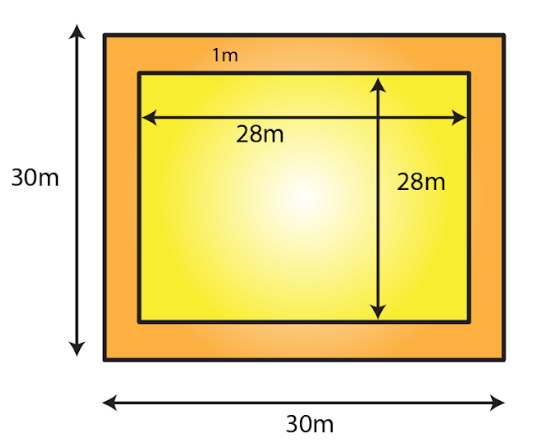Given that side of a square garden = 30m = a

We know that area of square = a2

Area of the square garden including the path = a2

= (30)2 = 900 m2

From the figure, it can be observed that the side of the square garden, when the path is not included, is 28 m.

Area of the square garden not including the path = (28) 2

= 784 m2

Total cost of covering the park with grass = Area of the park covering with green grass x Rate per square metre

1176 = 784 x Rate per square metre

Rate per square metre at which the park is covered with grass = (1176/784)

= Rs. 1.50 per m2

3. Through a rectangular field of sides 90 m x 60 m, two roads are constructed which are parallel to the sides and cut each other at right angles through the center of the field. If the width of the roads is 3 m, find the total area covered by the two roads.

Solution: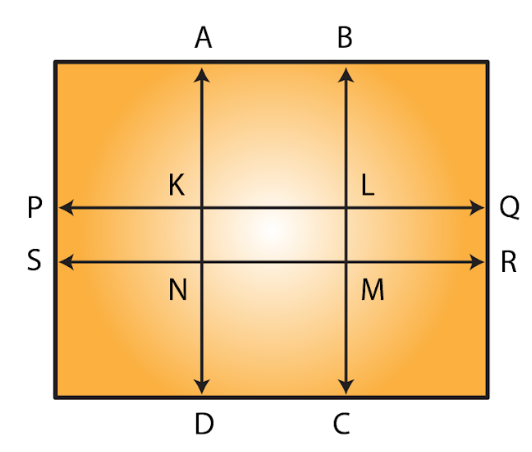Given length of rectangular field = 90m

Breadth of rectangular field = 60m

Area of the rectangular field = 90 m x 60 m = 5400 m2

Area of the road PQRS = 90 m x 3 m

= 270 m2

Area of the road ABCD = 60 m x 3 m

= 180 m2

Clearly, area of KLMN is common to the two roads.

Thus, area of KLMN = 3 m x 3 m

= 9 m2

Hence, area of the roads = Area (PQRS) + Area (ABCD) – Area (KLMN)

= (270 + 180) m2 – 9 m2

= 441 m2

4. From a rectangular sheet of tin, of size 100 cm by 80 cm, are cut four squares of side 10 cm from each corner. Find the area of the remaining sheet.

Solution: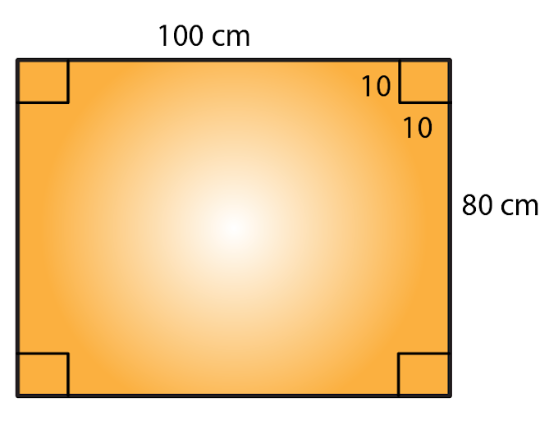Given that length of rectangular sheet = 100 cm

Breadth of rectangular sheet = 80 cm

Area of the rectangular sheet of tin = 100 cm x 80 cm

= 8000 c m2

Side of the square at the corner of the sheet = 10 cm

Area of one square at the corner of the sheet = (10 cm)2

= 100 cm2

Area of 4 squares at the corner of the sheet = 4 x 100 cm2

= 400 cm2

Hence, Area of the remaining sheet of tin = Area of the rectangular sheet – Area of the 4 squares

Area of the remaining sheet of tin = (8000 – 400) cm2

= 7600 cm2

5. A painting 8 cm long and 5 cm wide is painted on a cardboard such that there is a margin of 1.5 cm along each of its sides. Find the total area of the margin.

Solution: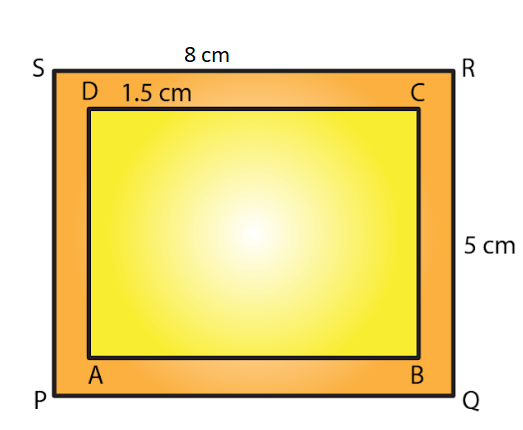Given length of cardboard including margin = 8 cm

Breadth of the cardboard including margin = 5 cm

Area of the cardboard including the margin = 8 cm x 5 cm = 40 c m2

From the figure, we can observed that,

New length of the painting without margin = 8 cm – (1.5 cm + 1.5 cm)

= (8 – 3) cm

= 5 cm

New breadth of the painting without margin = 5 cm – (1.5 cm + 1.5 cm)

= (5 – 3) cm

= 2 cm

Area of the painting not including the margin = 5 cm x 2 cm = 10 cm2

Hence, Area of the margin = Area of the cardboard including the margin – Area of the painting

= (40 – 10) cm2

= 30 cm2

6. Rakesh has a rectangular field of length 80 m and breadth 60 m. In it, he wants to make a garden 10 m long and 4 m broad at one of the corners and at another corner, he wants to grow flowers in two floor-beds each of size 4 m by 1.5 m. In the remaining part of the field, he wants to apply manures. Find the cost of applying the manures at the rate of Rs 300 per area.

Solution: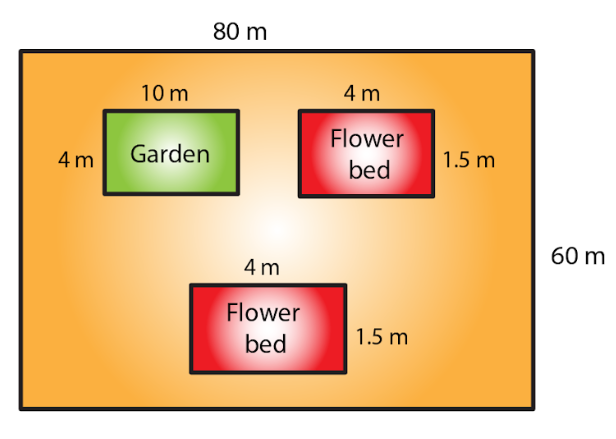Given that length of a rectangular field = 80 m

Breadth of a rectangular field = 60 m

Area of rectangular field = length x breadth

= (80 x 60) m

= 4800 m2

Again, Area of the garden = 10 m x 4 m = 40 m2

Area of one flower bed = 4 m x 1.5 m = 6 m2

Thus, Area of two flower beds = 2 x 6 m2 = 12 m2

Remaining area of the field for applying manure = Area of the rectangular field – (Area of the garden + Area of the two flower beds)

Remaining area of the field for applying manure = 4800 m2 – (40 + 12) m2

= (4800 – 52) m2

= 4748 m2

Since 100 m2 = 1 acre

Therefore by using the above

4748 m2 = 47.48 acres

So, cost of applying manure at the rate of Rs. 300 per are will be = Rs. (300 x 47.48)

= Rs. 14244

7. Each side of a square flower bed is 2 m 80 cm long. It is extended by digging a strip 30 cm wide all around it. Find the area of the enlarged flower bed and also the increase in the area of the flower bed.

Solution: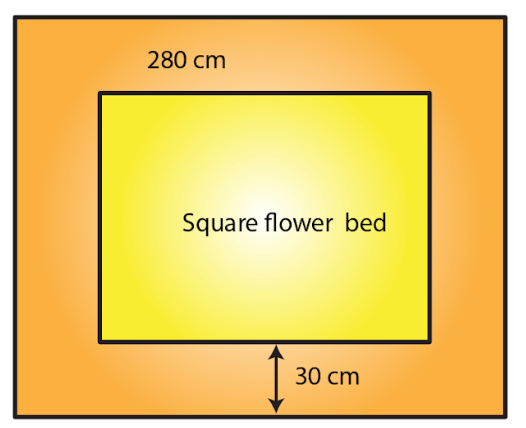Given side of the flower bed = 2 m 80 cm = 2.80 m [since 100cm = 1m]

Area of the square flower bed = (Side)2

= (2.80 m)2

= 7.84 m2

Side of the flower bed with strip = 2.80 m + 30 cm + 30 cm

= (2.80 + 0.3 + 0.3) m

= 3.4 m

Area of the enlarged flower bed with the digging strip = (Side)2

= (3.4)2

= 11.56 m2

Thus, Increase in the area of the flower bed = Area of the enlarged flower bed with the digging strip – Area of the square flower bed

= 11.56 m2 – 7.84 m2

= 3.72 m2

8. A room 5 m long and 4 m wide is surrounded by a verandah. If the verandah occupies an area of 22 m2, find the width of the verandah.

Solution: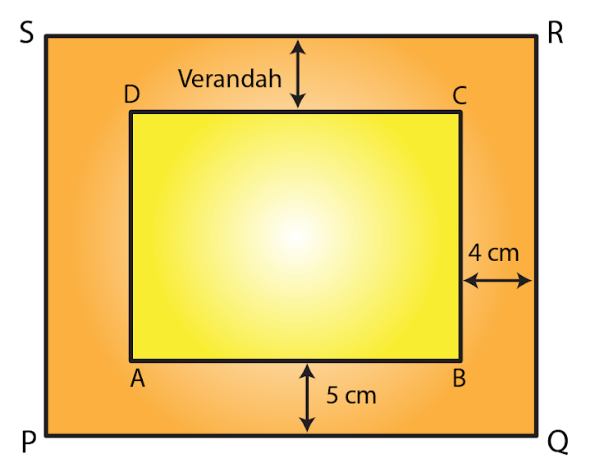Let the width of the verandah be x m.

Given Length of the room AB = 5 m and breadth of the room, BC = 4 m

We know that area of rectangle = length x breadth

Area of the room = 5 m x 4 m

= 20 m2

From the figure, it is clear that

Length of the veranda PQ = (5 + x + x) = (5 + 2x) m

Breadth of the veranda QR = (4 + x + x) = (4 + 2x) m

Area of veranda PQRS = (5 + 2x) x (4 + 2x)

= (4×2 + 18x + 20) m2

Area of veranda = Area of PQRS – Area of ABCD

22 = 4x2 + 18x + 20 – 20

22 = 4x2 + 18x

On dividing above equation by 2 we get,

11 = 2x2 + 9x

2x2 + 9x – 11 = 0

2x2 + 11x – 2x – 11 = 0

x (2x+11) – 1 (2x+11) = 0

(x- 1) (2x+11)= 0

When x – 1 = 0, x = 1

When 2x + 11 = 0, x = (-11/2)

The width cannot be a negative value. So, width of the veranda = x = 1 m

9. A square lawn has a 2 m wide path surrounding it. If the area of the path is 136 m2, find the area of the lawn.

Solution: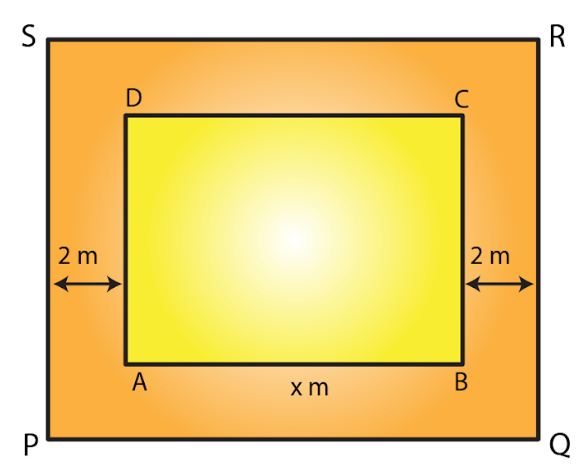Let ABCD be the square lawn and PQRS be the outer boundary of the square path.

Let side of the lawn AB be x m.

Area of the square lawn = x2

Given that Length PQ = (x + 2 + 2) = (x + 4) m

Area of PQRS = (x + 4)2 = (x2 + 8x + 16) m2

Now, Area of the path = Area of PQRS – Area of the square lawn

136 = x2 + 8x + 16 – x2

136 = 8x + 16

136 – 16 = 8x

120 = 8x

x = 120/ 8 = 15

Side of the lawn = 15 m

Hence, Area of the lawn = (Side)2

= (15 m)2

= 225 m2

10. A poster of size 10 cm by 8 cm is pasted on a sheet of cardboard such that there is a margin of width 1.75 cm along each side of the poster. Find (i) the total area of the margin (ii) the cost of the cardboard used at the rate of Re 0.60 per c m2.

Solution: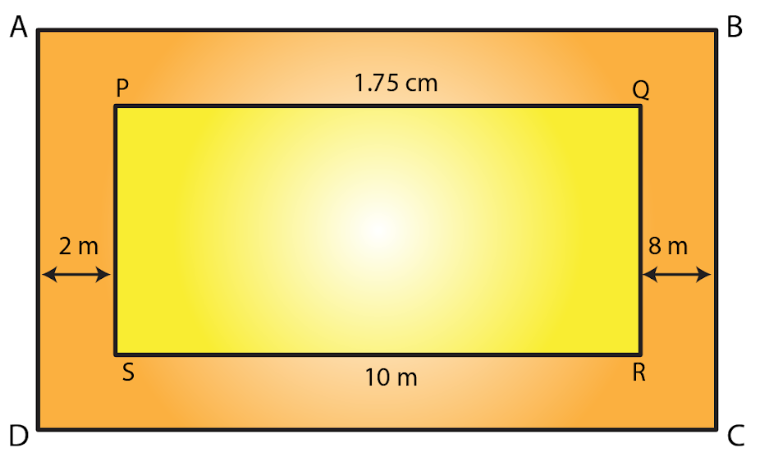Given length of poster = 10 cm and breadth of poster = 8 cm

Area of the poster = Length x Breadth

= 10 cm x 8 cm

= 80 cm2

From the figure, it is clear that

Length of the cardboard when the margin is included = 10 cm + 1.75 cm + 1.75 cm

= 13.5 cm

Breadth of the cardboard when the margin is included = 8 cm + 1.75 cm + 1.75 cm

= 11.5 cm

Area of the cardboard = Length x Breadth

= 13.5 cm x 11.5 cm

= 155.25 c m2

(i) Area of the margin = Area of cardboard including the margin – Area of the poster

= 155.25 c m2 – 80 c m2

= 75.25 c m2

(ii) Cost of the cardboard = Area of cardboard x Rate of the cardboard Rs 0.60 per cm2

= (155.25 x 0.60)

= Rs. 93.15

11. A rectangular field is 50 m by 40 m. It has two roads through its center, running parallel to its sides. The widths of the longer and shorter roads are 1.8 m and 2.5 m respectively. Find the area of the roads and the area of the remaining portion of the field.

Solution: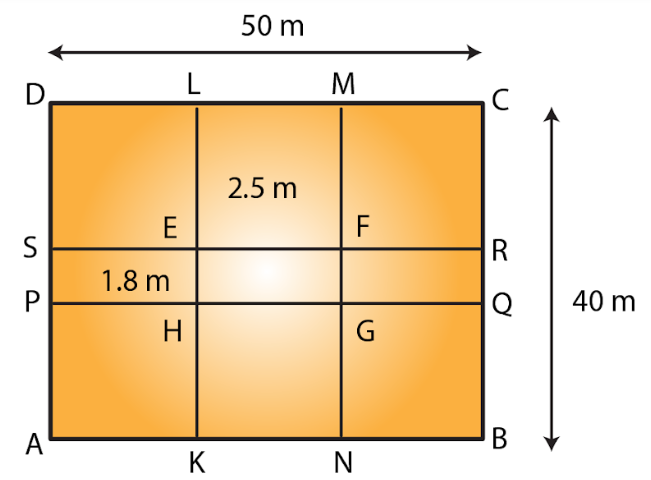Let ABCD be the rectangular field and KLMN and PQRS the two rectangular roads with width 1.8 m and 2.5 m, respectively.

Given that length of rectangular field = 50 m

Breadth of rectangular field = 40m

Area of rectangular field ABCD = 50 m x 40 m

= 2000 m2

Area of the road KLMN = 40 m x 2.5 m

= 100 m2

Area of the road PQRS = 50 m x 1.8 m

= 90 m2

Clearly area of EFGH is common to the two roads.

Thus, Area of EFGH = 2.5 m x 1.8 m = 4.5 m2

Hence, Area of the roads = Area of KLMN + Area of PQRS – Area of EFGH

= (100 m2 + 90 m2) – 4.5 m2

= 185.5 m2

Area of the remaining portion of the field = Area of the rectangular field ABCD – Area of the roads

= (2000 – 185.5) m2

= 1814.5 m2

12. There is a rectangular field of size 94 m x 32 m. Three roads each of 2 m width pass through the field such that two roads are parallel to the breadth of the field and the third is parallel to the length. Calculate: (i) area of the field covered by the three roads (ii) area of the field not covered by the roads.

Solution: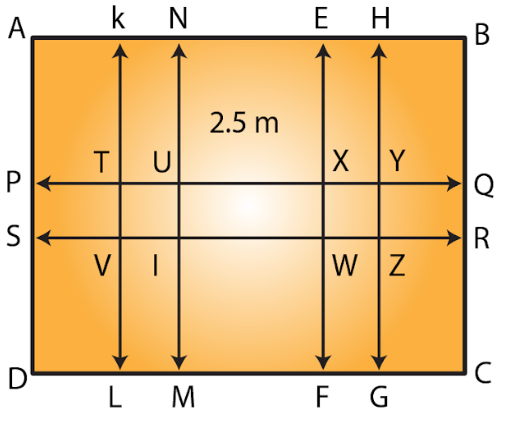Let ABCD be the rectangular field.

From the figure it is clear that two roads which are parallel to the breadth of the field KLMN and EFGH with width 2 m each.

One road which is parallel to the length of the field PQRS with width 2 m.

We have from the question, length of the rectangular field AB = 94 m and breadth of the rectangular field BC = 32 m

Area of the rectangular field = Length x Breadth

= 94 m x 32 m

= 3008 m2

Area of the road KLMN = 32 m x 2 m

= 64 m2

Area of the road EFGH = 32 m x 2 m

= 64 m2

Area of the road PQRS = 94 m x 2 m

= 188 m2

Clearly area of TUVI and WXYZ is common to these three roads.

Thus, Area of TUV1 = 2 m x 2 m = 4 m2

Area of WXYZ = 2 m x 2 m = 4 m2

(i) Area of the field covered by the three roads = Area of KLMN + Area of EFGH + Area (PQRS) – (Area of TUVI + Area of WXYZ)

= [64+ 64 + 188 – (4 + 4)] m2

= 316 m2 – 8 m2

= 308 m2

(ii) Area of the field not covered by the roads = Area of the rectangular field ABCD – Area of the field covered by the three roads

= 3008 m2 – 308 m2

= 2700 m2

13. A school has a hall which is 22 m long and 15.5 m broad. A carpet is laid inside the hall leaving all around a margin of 75 cm from the walls. Find the area of the carpet and the area of the strip left uncovered. If the width of the carpet is 82 cm, find the cost at the rate of Rs 18 per meter.

Solution: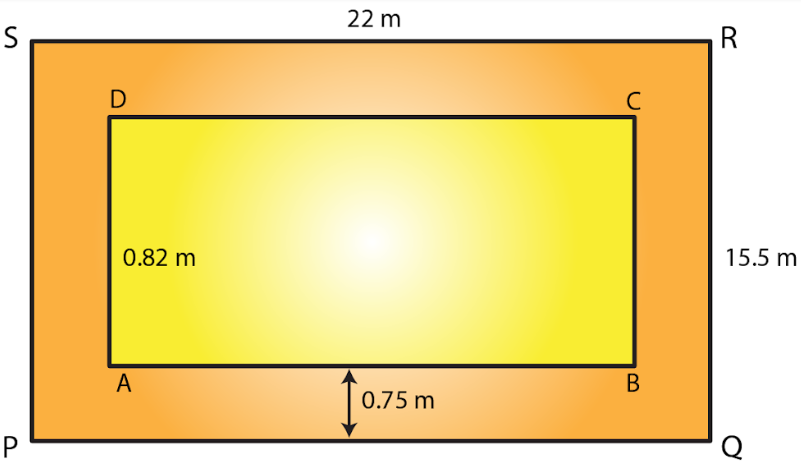Given that length of hall PQ = 22 m and breadth of hall QR = 15.5 m

Area of the school hall PQRS = 22 m x 15.5 m

= 341 m2

Length of the carpet AB = 22 m – (0.75 m + 0.75 m)

= 20.5 m [Since 100 cm = 1 m]

Breadth of the carpet BC = 15.5 m – (0.75 m + 0.75 m)

= 14 m

Area of the carpet ABCD = 20.5 m x 14 m

= 287 m2

Area of the strip = Area of the school hall PQRS – Area of the carpet ABCD

= 341 m2 – 287 m2

= 54 m2

Now, area of the 1 m length of carpet = 1 m x 0.82 m

= 0.82 m2

Thus, Length of the carpet whose area is 287 m2 = 287 m+ 0.82 m2

= 350 m

Cost of the 350 m long carpet = Rs. 18 x 350

= Rs. 6300

14. Two cross roads, each of width 5 m, run at right angles through the center of a rectangular park of length 70 m and breadth 45 m parallel to its sides. Find the area of the roads. Also, find the cost of constructing the roads at the rate of Rs 105 per m2.

Solution: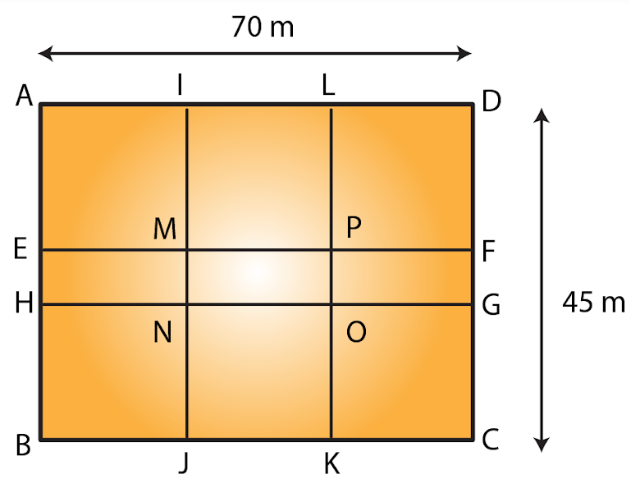Let ABCD be the rectangular park then EFGH and IJKL are the two rectangular roads with width 5m.

Given that length of rectangular park = 70m

Breadth of rectangular park = 45m

Area of the rectangular park = Length x Breadth

= 70 m x 45 m

= 3150 m2

Area of the road EFGH = 70 m x 5 m

= 350 m2

Now, Area of the road JKIL = 45 m x 5 m

= 225 m2

From the figure, it is clear that area of MNOP is common to the two roads.

Thus, Area of MNOP = 5 m x 5 m = 25 m2

Therefore,

Area of the roads = Area of EFGH + Area of JKIL – Area of MNOP

= (350 + 225) m2– 25 m2

= 550 m2

Again, it is given that the cost of constructing the roads = Rs. 105 per m2

Therefore,

Cost of constructing 550 m2 area of the roads

= Rs. (105 x 550)

= Rs. 57750.

15. The length and breadth of a rectangular park are in the ratio 5: 2. A 2.5 m wide path running all around the outside the park has an area 305 m2. Find the dimensions of the park.

Solution: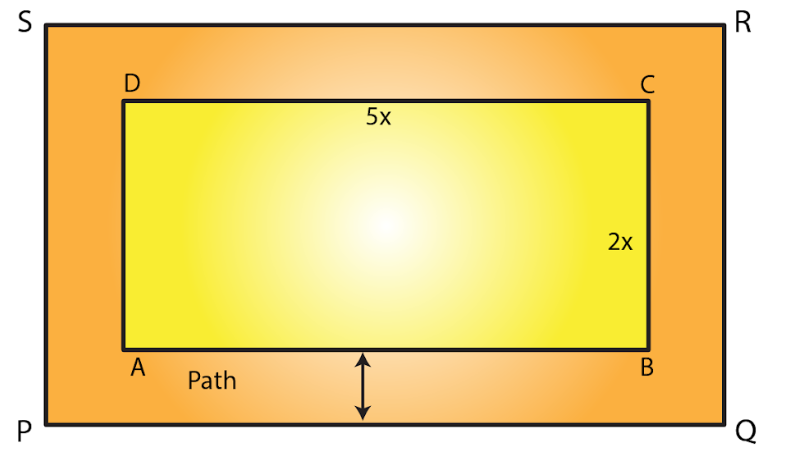Given that area of the park be 305m2.

Let the length of the park be 5x m and breadth be 2x m.

Therefore,

Area of the rectangular park = (5x) x (2x)

= 10x2 m2

Width of the path = 2.5 m

From the figure,

Outer length PQ = 5x m + 2.5 m + 2.5 m

= (5x + 5) m

Outer breadth QR = 2x + 2.5 m + 2.5 m

= (2x + 5) m

Area of PQRS = (5x + 5) m x (2x + 5) m

= (10x2 + 25x + 10x + 25) m2

= (10x2 + 35x + 25) m2

Area of the path = [(10x2 + 35x + 25) – 10x2] m2

By solving above equation we get

305 = 35x + 25

305 – 25 = 35x

280 = 35x

x = 280/35 = 8

Therefore,

Length of the park = 5x

= 5 x 8 = 40 m

Breadth of the park = 2x

= 2 x 8 = 16 m

16. A square lawn is surrounded by a path 2.5 m wide. If the area of the path is 165 m2, find the area of the lawn.

Solution: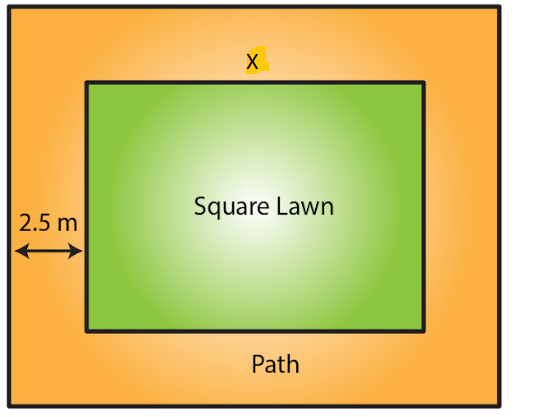Let the side of lawn be x m.

Given that width of the path = 2.5 m

Side of the lawn including the path = (x + 2.5 + 2.5) m

= (x + 5) m

Therefore, area of lawn = (Area of the lawn including the path) – (Area of the path)

We know that the area of a square = (Side)2

Area of lawn (x2) = (x + 5)2 – 165

x2 = (x2 + 10x + 25) – 165

165 = 10x + 25

165 – 25 =10x

140 = 10x

Therefore x = 140/10 = 14

Thus the side of the lawn = 14 m

Therefore,

The area of the lawn = (14 m) 2

= 196 m2